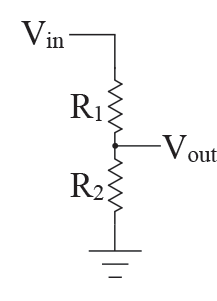# Finding component values of bandpass filter with load?I have to find the components of a bandpass filter given only the two corner frequencies. The only components are $C_1$ and $R_1$ for the high pass, and $R_2$, $C_2$, and Load resistance ($5$M$\Omega$) on the low pass.

I set $R_1$ to $10$k$\Omega$ and got $C_1 = 53.05$nF when corner frequency for high pass is $300$Hz using $C=1/2\pi fR_1$.

However, for the low pass... I can't figure it out. I combined $C_2$ and the load into equivalent impedance $Z$ (they are in parallel), then plugged $Z$ into the transfer equation for a low pass filter where ever a $C$ would have normally appeared, and get $C_2 = 1.588$nF while $R_2 = 10$k$\Omega$ (setting transfer of low pass filter equal to $1/\sqrt{2}$). Also corner frequency of low pass filter is 10kHz. But the graph of the output voltage looks like a high pass filter only, and is in the micro volts and the -3db frequencies are nowhere even close... I don't get what is going wrong with the low pass filter

So was wondering if anyone can show me how to calculate the $R$ and $C$ values of the low pass filter part of a bandpass filter when it is connected to a load?

It is quite a basic bandpass filter, but imagine a 5Mohm load where Vout is.

• You should really post a schematic of the circuit you are trying to analyze/design. Feb 17, 2015 at 2:29

Your low pass filter is loading your high pass filter. Think of a simple voltage divider:. In general, if you have 2 circuits connected together, the first one's output to the second one's input, you do not want the second one to attenuate the signal that passes through the first one. Therefore, you must make sure that the second circuit's input impedance (represented by R2 in the voltage divider) is larger than your first circuit's output impedance. How much larger? The rule of thumb is 10x larger.
• Yes, that's right, you will get the cutoff frequency of the low pass if you do that. As an exercise, I would try to get the transfer function of the entire circuit and set it equal to $\frac{1}{\sqrt{2}}$ to get both cutoff frequencies. If you need help with deriving the transfer function, see this question: electronics.stackexchange.com/questions/152159/… Feb 17, 2015 at 15:14
• To clarify, if the input impedance of the second stage is approximately 10 times the output impedance of the first stage, the total transfer function $H(\omega)$ is approximately $H_2(\omega) \times H_2(\omega)$, the product of the individual transfer functions of both stages. Therefore, you don't have to calculate $H(\omega)$ in order to construct your BPF - you just need $H_1(\omega)$ and $H_2(\omega)$. Feb 18, 2015 at 3:16